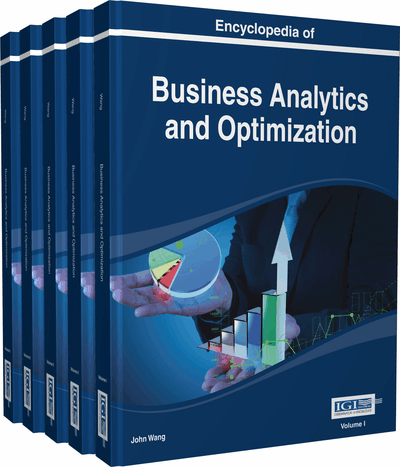# Input Analysis for Stochastic Simulations

David Fernando Muñoz (Instituto Tecnológico Autónomo de México, México)
DOI: 10.4018/978-1-4666-5202-6.ch112

Top

## Introduction

Simulation has been recognized as a powerful tool for forecasting, risk assessment, animation and illustration of the evolution of a system in many areas (see, e.g., Kelton et al., 2011), including business analytics. When uncertainty on the behavior of some components of the simulation model is present, these random components of a stochastic simulation are modeled through the use of probability distributions and/or stochastic processes that drive the simulation experiments.

(1)

The congestion at the ATM can be simulated by using Equation (1) (e.g., on a spreadsheet) to produce clients’ waiting times until the last client’s departure exceeds time. However, in order to produce the simulation output given by Equation (1), we need to produce two streams of random inputs:, and. These streams are usually (although not always) produced by assuming that the inter-arrival times are independent and identically distributed (i.i.d.) random variables from a density function, and the service times are i.i.d. random variables from a density function, whereandare known parameters. Then, standard methods for random variate generation (see, e.g., Law 2007) can be applied to obtain the required streams, and.

## Key Terms in this Chapter

Simulation Output Analysis: Statistical analysis of the output from simulation experiments.

Distribution Fitting: The fitting of a probability distribution to a data set with the goal of finding the distribution that best model the random pattern in the data set.

Parameter Uncertainty: The lack of certainty on the value of the parameters of a forecasting model.

Data Analysis: The process of inspecting, cleaning, transforming, and modeling data with the goal of extracting useful information.

Parameter Estimation: The process of approximating the unknown parameters of a probability distribution from observations.

Forecasting Using Simulation: Using simulation techniques to make statements about events whose actual outcomes have not yet been observed.

Simulation Input Analysis: The modeling of random inputs in a stochastic simulation.

Bayesian Forecasting: Using Bayesian statistics to make statements about events whose actual outcomes have not yet been observed.

## Complete Chapter List

Search this Book:
Reset